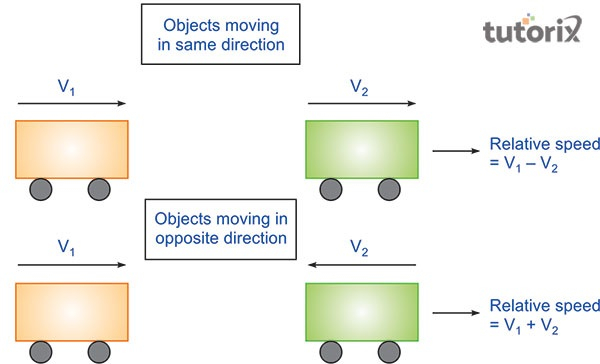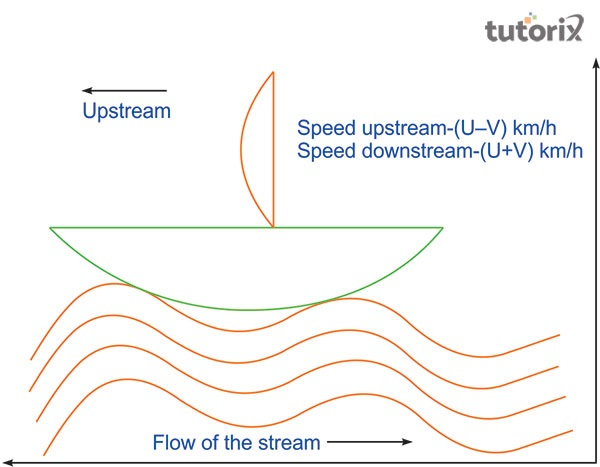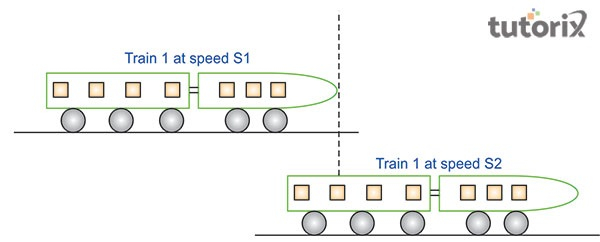# Relative Speed

## Introduction

Relative speed is calculated by assessing the difference between two moving things that are travelling in the same direction. When moving things travel in the opposite direction, the speed of both the objects is calculated to compute the relative speed of the objects that are moving in different directions.

## What is Relative Speed?

The idea of relative speed is applied when two or more bodies (objects) travel at a certain speed in the same or different directions. Therefore, relative speed can be defined as a concept that calculates the speed of one moving body concerning another body, travelling at the same speed.

For example, if two people are running in the opposite direction, the relative speed of one person can be calculated about the other stationary body, as the bodies are travelling in different directions at the same time. So, relative speed can be described as the speed of a moving body concerning another stationary body.

## Ways to calculate Relative Speed

The calculation of relative speed in the case of bodies moving in the same direction and bodies moving in the opposite direction are different. While calculating the relative speed of bodies that are moving in the same direction, the difference between the bodies is calculated. On the other hand, the speed of different bodies is added while calculating the relative speed of objects that are travelling in the opposite direction from each other (Chen et al. 2021).

For example, if the speed of the first body s x km/h and the speed of the second body is y km/h, then the relative speed of the bodies here is going to be ${(x\: -\: y) \:km/h\: [x \:\gt\: y]}$Figure 1: Relative speed

The formula of distance travelled/relative speed is applied while calculating the time between the bodies, when they meet. For example, for body x and body y, the time will be $dkm/(x\: - \:y) \:km/h$

Time is signified with t while calculating the elapsed time, taken by two bodies while travelling at a certain relative speed (Suresolv, 2022).

## Common problems found in the calculation of Relative Speed

The calculation of relative speed can be segmented into three types of problems; the first one is related to the relative speed of boats upstream and downstream.

• The second one is related to the travelling time and meeting time of trains based on their relative speed. The third one is based on the ratio-based issues while calculating the relative speed of moving bodies or objects (Murray, Gabbett & Townshend, 2018).

### Problems related to Boats and Streams

In the case of boats and streams, the speed of the river is the medium and the speed of the boat is not constant at all. So, when the water is steady or it has a certain flow, it depends on two different conditions, both in the case of the boat and the stream.Figure 2: Upstream and Downstream in Boats

According to the above picture, when the boat moves against the flow of the stream it moves in the upstream and then it goes along with the stream, it stays the downstream. Based on these two conditions, the relative speed of the boat differs. For calculating the upstream, the $(u \:- \:v) \:km/h$ is applied whereas for downstream, the formula is $(u \:+\: v)\: km/h$ (Physicstutorials, 2022).

### Meeting Time and Travel time problems

In the case of this type of relative speed problem, it requires two moving things travelling in the same or the opposite direction with a certain speed. Here, when two objects start their movement towards each other with the same amount of speed, the meeting point of the objects should also be the same (Chen et al. 2021).Figure 3: Time and Distance in trains

Here, the covered distance is proportional to each other. For example, in the above diagram, two trains (S1 and S2) are travelling in the same direction at the same speed. Therefore, when these two trains meet at a certain point, it should cost them the same amount of time to reach the meeting point.

### Ratio-based problems

Ratio-based problems can be pointed out when two objects are moving in the same direction with the same speed. For example, if object A is travelling towards object B with the same amount of speed and vice versa, the ratio of speed for both the objects is going to be A: B. Here, the time of reaching each other may differ but the speed is going to be constant for both the objects.

## Conclusion

Relative speed is the concept of calculating the speed of two objects that are moving in the same or the opposite direction with a certain amount of speed. Time plays a crucial role in the calculator of the relative speed of persons or objects that are moving towards or opposite each other. Calculating relative speed follows problems like boats and streams, time and distance of trains and ratio-based problems while assessing the relative speed of objects moving in the same or opposite direction from each other.

## FAQs

Q1. How does the concept of relative speed work?

Ans. Relative speed work as the rate of two moving objects or bodies going in the same or opposite direction from each other. It works by calculating the difference and time under different conditions.

Q2. What is the basic difference between average speed and relative speed?

Ans. The average speed is the speed of the whole journey of two objects or bodies. The relative speed is the difference of speed moving in the same or opposite direction from each other.

Q3. What is the formula for calculating time with relative speed?

The formula is t = relative speed * time. Here, the visual representation of the formula is like, $(x\: -\: y)km /h * t$